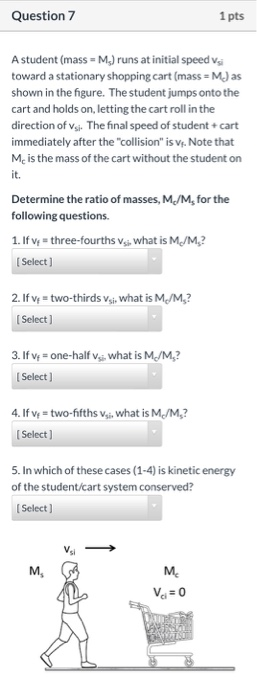# Question A student (mass = Ms) runs at initial speed vsi toward a stationary shopping cart (mass = Mc) as shown in the figure. The student jumps onto the cart and holds on, letting the cart roll in the direction of vsi. The final speed of student + cart immediately after the "collision" is vf. Note that Mc is the mass of the cart without the student on it.Determine Question 7 1pts A student (mass-M.) runs at initial speed v toward a stationary shopping cart (mass = M.J as shown in the figure. The student jumps onto the cart and holds on, letting the cart roll in the direction of vsi. The final speed of student+ cart immediately after the "collision" is v,. Note that Mc is the mass of the cart without the student on it Determine the ratio of masses, M/M, for the following questions 1. If v three-fourths vs. what is M/M,? [ Select 2. If v = two-thirds v j. what is M/M,? Select] 3. If v = one-halfv. what is M./M,? Select] 4. If v = two-fifths v. what is M,/M,? Select 5. In which of these cases (1-4) is kinetic energy of the student/cart system conserved? Select] м, м. Ve= 0